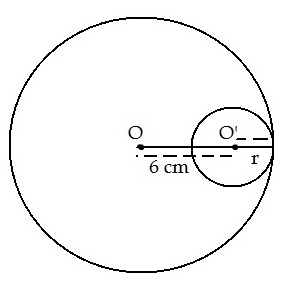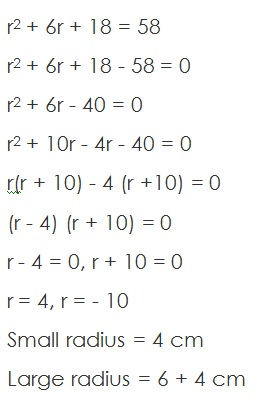Study Materials: CBSE Notes

Our CBSE Notes for Additional -Questions With Solutions Class 10 maths 12. Areas Related to Circles - CBSE Notes - Toppers Study is the best material for English Medium students cbse board and other state boards students.

Notes ⇒ Class 10th ⇒ Mathematics ⇒ 12. Areas Related to Circles

# Additional -Questions With Solutions Class 10 maths 12. Areas Related to Circles - CBSE Notes - Toppers Study

Topper Study classes prepares CBSE Notes on practical base problems and comes out with the best result that helps the students and teachers as well as tutors and so many ecademic coaching classes that they need in practical life. Our CBSE Notes for Additional -Questions With Solutions Class 10 maths 12. Areas Related to Circles - CBSE Notes - Toppers Study is the best material for English Medium students cbse board and other state boards students.

## Additional -Questions With Solutions Class 10 maths 12. Areas Related to Circles - CBSE Notes - Toppers Study

CBSE board students who preparing for class 10 ncert solutions maths and Mathematics solved exercise chapter 12. Areas Related to Circles available and this helps in upcoming exams 2023-2024.

### You can Find Mathematics solution Class 10 Chapter 12. Areas Related to Circles

• All Chapter review quick revision notes for chapter 12. Areas Related to Circles Class 10
• NCERT Solutions And Textual questions Answers Class 10 Mathematics
• Extra NCERT Book questions Answers Class 10 Mathematics
• Importatnt key points with additional Assignment and questions bank solved.

NCERT Solutions do not only help you to cover your syllabus but also will give to textual support in exams 2023-2024 to complete exercise - maths class 10 chapter 12 in english medium. So revise and practice these all cbse study materials like class 10 maths chapter - in english ncert book. Also ensure to repractice all syllabus within time or before board exams for ncert class 10 maths ex - in english.

See all solutions for class 10 maths chapter 12 exercise 12 in english medium solved questions with answers.

### Additional -Questions With Solutions class 10 Mathematics Chapter 12. Areas Related to Circles

• Additional -Questions With Solutions Class 10 Maths 12. Areas Related To Circles - CBSE Notes - Toppers Study
• Class 10 Ncert Solutions
• Solution Chapter 12. Areas Related To Circles Class 10
• Solutions Class 10
• Chapter 12. Areas Related To Circles Additional -Questions With Solutions Class 10

## Additional -Questions With Solutions Class 10 maths 12. Areas Related to Circles - CBSE Notes - Toppers Study

Q1. Two circles touch internally, the sum of their areas is 116 π. Distance between their center is 6 cm. Find the radii of circles.

Solution:Let the radius of small circle = r cm.

Then the radius of Large circle R = 6 + r cm.

Sum of their areas = 116π cm

πR2 + πr2 = 116 π cm

π(R2+ r2) = 116π

R2+ r2 = 116

(6 + r)2 + r2 = 116

36 + r2 + 12r + r= 116

2r2 + 12r + 36 = 116##### Other Pages of this Chapter: 12. Areas Related to Circles

Important Study materials for classes 06, 07, 08,09,10, 11 and 12. Like CBSE Notes, Notes for Science, Notes for maths, Notes for Social Science, Notes for Accountancy, Notes for Economics, Notes for political Science, Noes for History, Notes For Bussiness Study, Physical Educations, Sample Papers, Test Papers, Mock Test Papers, Support Materials and Books.Mathematics Class - 11th

NCERT Maths book for CBSE Students.

books

## Study Materials List:

##### Notes ⇒ Class 10th ⇒ Mathematics
1. Real Numbers
2. Polynomials
3. Pair of Linear Equations in Two Variables
5. Arithmetic Progressions
6. Triangles
7. Coordinate Geometry
8. Introduction to Trigonometry
9. Some Applications of Trigonometry
10. Circles
11. Constructions
12. Areas Related to Circles
13. Surface Areas and Volumes
14. Statistics
15. Probability

New Books